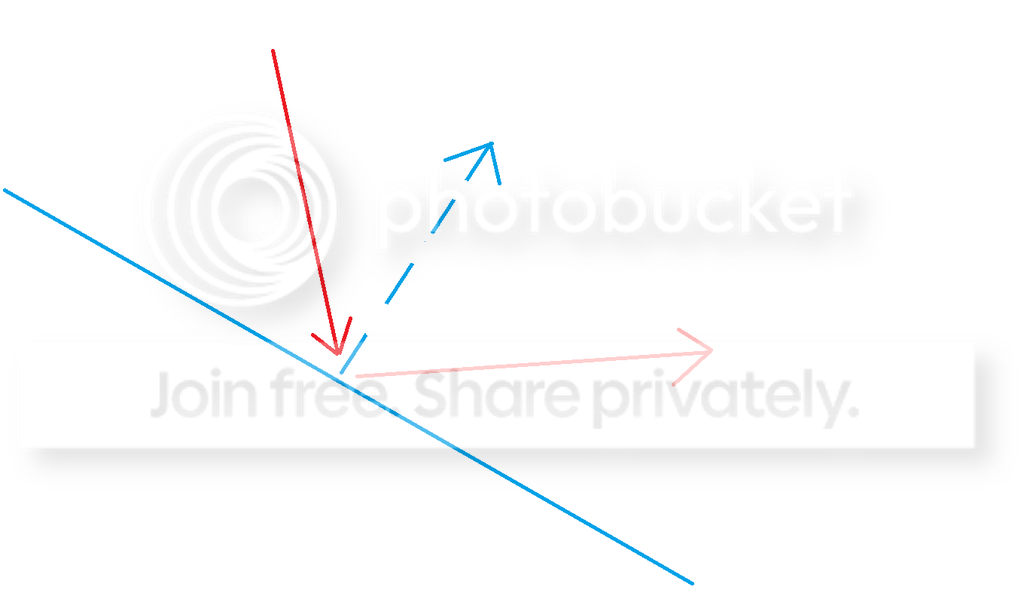I apologize for asking the same thing here. The forum becomes depopulated and I 'm not an informatician, so I suck at maths.

It's about a ball new direction after bouncing.
The ball is a kinematic body 2D. I want the new vector direction to be symmetric to the previous direction for the sake of predictability. The axis of symmetry is the collision normal.
Like a breakout but with a formula who works for every collision normal.in Engine
recategorized
I'll move it to the Engine category as finally the best answer is Godot-specific :)

To find a reflection vector, you can use Vector2D/3D built-in function Vector2D reflect(Vector2D normal). I'm pretty sure the input vector is the reflection normal.

by (830 points)
selected by
That's it ! I I tried this function before asking here, but I used the wrong order. The doc description is a bit confusing, it talks about sliding.
Anyway, I guess using a built-in function is faster at runtime than write it "manually" in GDScript. Thanks.
–1 vote

This isn't really a Godot question and it can be solved super easily by googling "reflection vector".

First result

by (372 points)

What you are looking for is Vector2.reflect(). In order to give you a better idea of how you implement this, a full example of a BALL script (taken from this video).

Example of use:

Code in ball.gd that is attached to a KinematicBody2D.

Ball Scene Hierarchy:
KinematicBody2D [script attached here]
- Sprite
- CollisionShape2D
- VisibilityNotifier2D

GDScript:

``````extends KinematicBody2D

# Gravity
var _gravity = 0

# Movement
var _movement = Vector2()

# Bounce reduction
var bounce = 0.6

# Initialize shot from another script after adding it to the scene
func shoot(directional_force, gravity):
_movement = directional_force
_gravity = gravity
set_fixed_process(true)

# Processing - 60 ticks per second
func _fixed_process(delta):
# Simulate gravity
_movement.y += delta * _gravity

# Check if we have collided
if(is_colliding()):
# Get node that we are colliding with
var entity = get_collider()

# Apply physics
var normal = get_collision_normal()

# Apply bounce physics
# _movement = (_movement - 2 * _movement.dot(normal) * normal) * bounce
_movement = normal.reflect(_movement) * bounce

# Move
move(_movement)

# On screen exit
func _on_visibility_notifier_exit_screen():
queue_free()
``````

Note that in order to reflect the movement, you will need to assign it to the movement var that you want to "move" to.
*
movement = normal.reflect(_movement)*

In a nutshell:
NewMovementVector2 = NormalVector2.reflect(CurrentMovementVector2)

(I know this have technically been answered, but I figure an example of how it could be implemented would be very useful to majority of people) :)

by (495 points)